## A 2.0 kg block on a horizontal frictionless surface is attached to a spring whose force constant is 590 N/m. The block is pulled from its eq

Question

A 2.0 kg block on a horizontal frictionless surface is attached to a spring whose force constant is 590 N/m. The block is pulled from its equilibrium position at x = 0 m to a displacement x = +0.080 m and is released from rest. The block then executes simple harmonic motion along the x-axis (horizontal). The velocity of the block at time t = 0.10 s is closest to:________.

in progress 0
7 months 2021-08-05T21:42:48+00:00 1 Answers 2 views 0

## Answers ( )

The  value is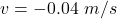Explanation:

From the question we are told that

The  mass  of the block is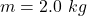The  force constant  of the spring is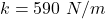The amplitude  is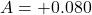The  time consider is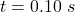Generally the angular velocity of this  block is mathematically represented as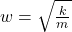=>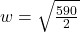=>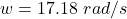Given that the block undergoes simple harmonic motion the velocity is mathematically represented as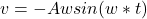=>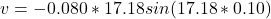=>